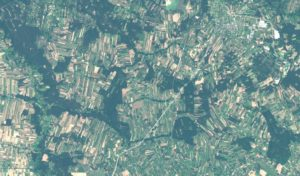Reading and writing georeferenced rasters in R is done using the raster library. We install the library using the code line:

`install.packages(‘raster’)`

After installation, you must initialize (activate) it so that the functions it contains are available:

`library(raster)`

For this exercise, we downloaded an image consisting of 4 channels (blue, green, red and near infrared) from Sentinel 2, which is available for free. Each of the channels is stored in a different file, which needs to be loaded into R. Use the grid function.

`b2 = raster('F:/B02.tif') `

`b3 = raster('F:/B03.tif') `

`b4 = raster('F:/B04.tif') `

`b8 = raster('F:/B08.tif')`

We can display basic information about each of them by typing the name of the variable they were written to in the console:

`> b2`

`class       : RasterLayer `

`dimensions  : 10980, 10980, 120560400  (nrow, ncol, ncell)`

`resolution : 10, 10 (x, y) `

`extent : 399960, 509760, 5690220, 5800020 (xmin, xmax, ymin, ymax)  `

`coord. ref. : +proj=utm +zone=34 +datum=WGS84 +units=m +no_defs +ellps=WGS84 +towgs84=0,0,0 `

`data source : F:\B02.tif `

`names       : B02 `

`values      : 0, 15810  (min, max)`

Remote sensing data usually consists of multiple channels stored in one or more files (in separate files in the example) . The function (structure) stack is used to combine channels and read raster data from multiple channels. As variables of the function we add the set of channels we want to store under one variable.

`b_all = stack(b2,b3,b4,b8)`

After displaying in the console variable b_all

`> b_all`

`class       : RasterStack `

`dimensions  : 10980, 10980, 120560400, 4  (nrow, ncol, ncell, nlayers)`

`resolution  : 10, 10  (x, y)`

`extent      : 399960, 509760, 5690220, 5800020  (xmin, xmax, ymin, ymax)`

`coord. ref. : +proj=utm +zone=34 +datum=WGS84 +units=m +no_defs +ellps=WGS84 +towgs84=0,0,0 `

`names       :   B02,   B03,   B04,   B08 `

`min values : 0, 0, 0, 0 `

`max values : 15810, 18613, 28000, 25910   `

You can display information about each channel. When loading a file with many channels, we also use this function, except that we specify the path to the file as an argument:

`b_all = stack('F:/B_ALL.tif')`

In variable b_all, which is a list of raster files, we still have the option to extract individual channels with:

`> b_all[]`

`class : RasterLayer dimensions : 10980, 10980, 120560400 (nrow, ncol, ncell) `

`resolution : 10, 10 (x, y) `

`extent : 399960, 509760, 5690220, 5800020 (xmin, xmax, ymin, ymax) `

`coord. ref. : +proj=utm +zone=34 +datum=WGS84 +units=m +no_defs +ellps=WGS84 +towgs84=0,0,0 `

`data source : F:\B02.tif `

`names : B02 `

`values : 0, 15810 (min, max)`

Save the raster file with the function writeRaster(‘variable name’, filename = ‘save path’, format = ‘save format’):

`writeRaster(b_all, filename = "F:/B_all.tif", format = "GTiff")`

You can preview the formats to which you can write raster data by typing:

`writeFormats()`

The saved GeoTIFF file contains 4 channels that can be used to create color compositions: